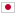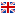Contest Duration: - (local time) (300 minutes)
D - Revenge of the Broken Door/Time Limit: 10 sec / Memory Limit: 512 MB

### Problem Statement

The JAG Kingdom consists of $N$ cities and $M$ bidirectional roads. The $i$-th road $(u_i,v_i,c_i)$ connects the city $u_i$ and the city $v_i$ with the length $c_i$. One day, you, a citizen of the JAG Kingdom, decided to go to the city $T$ from the city $S$. However, you know that one of the roads in the JAG Kingdom is currently under construction and you cannot pass the road. You don't know which road it is. You can know whether a road is under construction only when you are in either city connected by the road.

Your task is to minimize the total length of the route in the worst case. You don't need to decide a route in advance of departure and you can choose where to go next at any time. If you cannot reach the city $T$ in the worst case, output -1.

### Input

The input consists of a single test case formatted as follows.

$N$ $M$ $S$ $T$ $u_1$ $v_1$ $c_1$ $\vdots$ $u_M$ $v_M$ $c_M$

The first line contains four integers $N$, $M$, $S$, and $T$, where $N$ is the number of the cities ($2 \leq N \leq 100{,}000$), $M$ is the number of the bidirectional roads ($1 \leq M \leq 200{,}000$), $S$ is the city you start from ($1 \le S \le N$), and $T$ is the city you want to reach to ($1 \le T \le N$, $S \neq T$). The following $M$ lines represent road information: the $i$-th line of the $M$ lines consists of three integers $u_i$, $v_i$, $c_i$, which means the $i$-th road connects the cities $u_i$ and $v_i$ ($1 \leq u_i, v_i, \leq N$, $u_i \neq v_i$) with the length $c_i$ ($1 \leq c_i \leq 10^9$). You can assume that all the pairs of the cities are connected if no road is under construction. That is, there is at least one route from city $x$ to city $y$ with given roads, for all cities $x$ and $y$. It is also guaranteed that there are no multiple-edges, i.e., $\{u_i, v_i\} \neq \{u_j, v_j\}$ for all $1 \le i < j \le M$.

### Output

Output the minimum total length of the route in the worst case. If you cannot reach the city $T$ in the worst case, output -1.

### Sample Input 1

3 3 1 3
1 2 1
2 3 5
1 3 3


### Output for Sample Input 1

6


### Sample Input 2

4 4 1 4
1 2 1
2 4 1
1 3 1
3 4 1


### Output for Sample Input 2

4


### Sample Input 3

5 4 4 1
1 2 3
2 3 4
3 4 5
4 5 6


### Output for Sample Input 3

-1